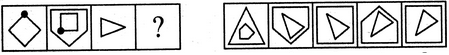# Non Verbal Reasoning - Analogy - Discussion

### Discussion :: Analogy - Section 1 (Q.No.26)

Each of the following questions consists of two sets of figures. Figures A, B, C and D constitute the Problem Set while figures 1, 2, 3, 4 and 5 constitute the Answer Set. There is a definite relationship between figures A and B. Establish a similar relationship between figures C and D by selecting a suitable figure from the Answer Set that would replace the question mark (?) in fig. (D).

26.

Select a suitable figure from the Answer Figures that would replace the question mark (?).

Problem Figures:                            Answer Figures:(A)     (B)      (C)     (D)                  (1)      (2)      (3)      (4)      (5)

 [A]. 1 [B]. 2 [C]. 3 [D]. 4 [E]. 5

Explanation:

The figure rotates through 135oACW and is placed inside another figure with one more number of sides.

 Raghu Mahajan said: (Sep 1, 2016) From a to b, figure rotates 90 degrees, not 135 degrees. So, this solution is wrong.

 Ranjit said: (Mar 2, 2018) How it works? Please explain me.

 Shanthi said: (May 14, 2018) Please explain me to get it.

 Anomii said: (Jul 1, 2018) Logically, 2 should also work because the other figure could just be consistently set to the second figure shown in B. Both options 2 and 3 should theoretically work.